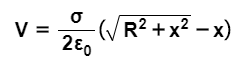# Problem: A disk with radius R has uniform surface charge density σ.By regarding the disk as a series of thin concentric rings, the electric potential V at a point on the disk's axis a distance x from the center of the disk is  Derive an expression for −∂V/∂x.Express your answer in terms of the given quantities and appropriate constants.

###### FREE Expert Solution

$\begin{array}{rcl}\mathbf{-}\frac{\mathbf{dV}}{\mathbf{dx}}& \mathbf{=}& \mathbf{-}\frac{\mathbf{d}}{\mathbf{d}\mathbf{x}}\mathbf{\left(}\frac{\mathbf{\sigma }}{\mathbf{2}{\mathbf{\epsilon }}_{\mathbf{0}}}\mathbf{\left(}\sqrt{{\mathbf{R}}^{\mathbf{2}}\mathbf{+}{\mathbf{x}}^{\mathbf{2}}}\mathbf{-}\mathbf{x}\mathbf{\right)}\mathbf{\right)}\\ & \mathbf{=}& \mathbf{-}\frac{\mathbf{\sigma }}{\mathbf{2}{\mathbf{\epsilon }}_{\mathbf{0}}}\frac{\mathbf{d}\mathbf{\left(}\sqrt{{\mathbf{R}}^{\mathbf{2}}\mathbf{+}{\mathbf{x}}^{\mathbf{2}}}\mathbf{-}\mathbf{x}\mathbf{\right)}}{\mathbf{d}\mathbf{x}}\end{array}$

79% (293 ratings)###### Problem Details

A disk with radius R has uniform surface charge density σ.

By regarding the disk as a series of thin concentric rings, the electric potential V at a point on the disk's axis a distance x from the center of the disk isDerive an expression for −∂V/∂x.

Express your answer in terms of the given quantities and appropriate constants.